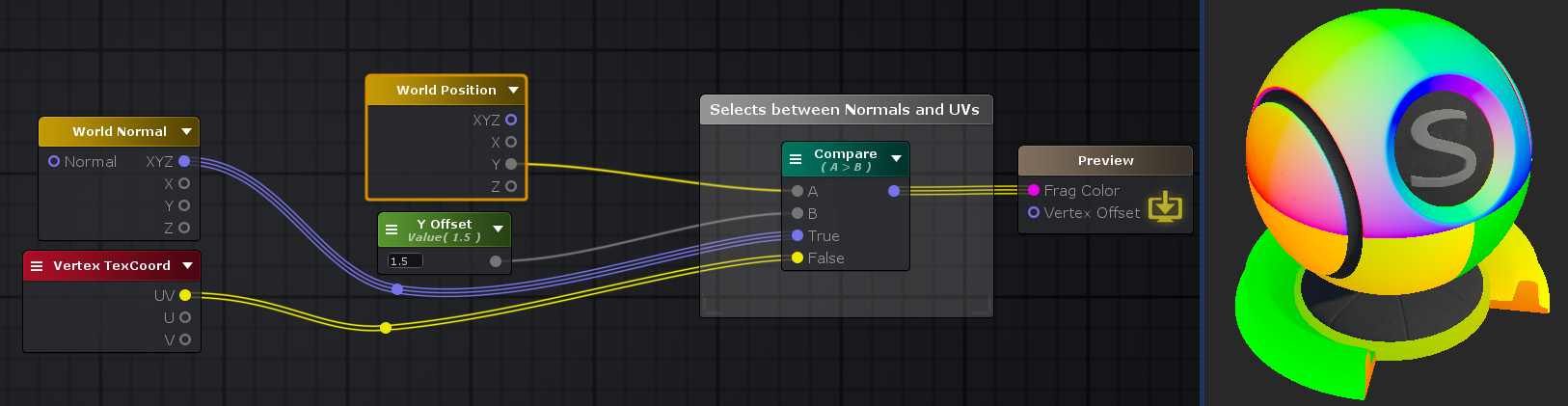## Compare Node

The Compare node compares two float inputs A and B and outputs a value from on one of its inputs True or False according to the comparison result.Nodes used: World Position, Float, World Normal, Vertex TexCoord, Compare

Node Parameter Description Default Value
Compare Op Selects compare operation to apply to inputs
• Equal: Outputs value connected to True if A equals B, else outputs value connected to False
• Not Equal: Outputs value connected to True if A is not equal to B, else outputs value connected to False
• Greater: Outputs value connected to True if A is greater than B, else outputs value connected to False
• Greater Or Equal: Outputs value connected to True if A greater or equal than B, else outputs value connected to False
• Less: Outputs value connected to True if A is less than B, else outputs value connected to False
• Less Or Equal: Outputs value connected to True if A is less or equal than B, else outputs value connected to False
Equal
A First value of the comparison operation. Only visible if the respective input port is not connected. 0
B Second value of the comparison operation. Only visible if the respective input port is not connected. 0

Input Port Description Type
A First value of the comparison operation. Float
B Second value of the comparison operation. Float
True Value to be outputted if comparison result is true. Float 
False Value to be outputted if comparison result is false. Float 

1. ^ Port automatically adapts to all connection types except Sampler types.